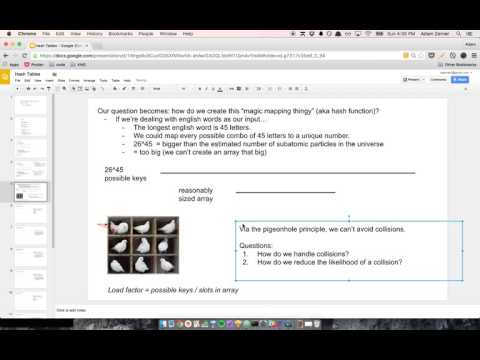# Big O, little n

I remember when I was first learning about hash tables. I thought I stumbled across an ingenious optimization for dealing with hash collisions. Before explaining my optimization, let me first briefly explain how hash tables work and what hash collisions are. I also made a video if you're interested.Say that we have a hash that maps someone's name to their age. `"adam"` is `27`, so we want to enter that into our hash.Here's what happens:

• `"adam"` gets put through a hash function.
• The hash function spits out `7`.
• `7` is the index of the array where we are going to store `"adam"`'s value.
• So we store `27` into `array`.

If we run `hash.get("adam")` in the future and need to look up the value for `"adam"`, we:

• Run `"adam"` through the hash function.
• Get `7` as the output.
• This means we have to look at `array` to get the value.
• So we look at `array` to get our value.

Hopefully if we have a key of `"alice"` or `"bob"` it'll hash to a different index. But... what happens if it hashes to the same index? That's called a hash collision.A common approach is that if there's a collision, you store the values in a linked list.Fair enough. That makes sense.

But wait!!! It takes `O(n)` time to look up a value in a linked list. Can't we use a binary search tree to speed that up to `O(log n)`???Or... can't we take it a step further and use a hash inside of a hash to get the lookup time all the way down to `O(1)`?!I hate to say it, but however many years ago when I had these thoughts, there were a few moments where I thought I was a genius. Now when I look back at that former self, I facepalm.

It is true that going from a linked list to a BST to a hash takes you from `O(n)` to `O(logn)` to `O(1)` lookup time. However, since collisions are rare, `n` is going to be small. And when `n` is small, `O(n)` might be faster than `O(1)`.

To understand why that is, think back to what Big-O really means. I think it helps to think about it as a "math thing" instead of a "programming thing". Consider two functions:

```f(n) = 4n + 1000
g(n) = 2n^2 + 5
```

Big-O of `f` is `O(n)` and Big-O of `g` is `O(n^2)`. We just focus on the part that "really matters". When `n` gets really big, the fact that it's `4n` doesn't really matter. Nor does the `+ 1000`.

But what about when `n` is small? Well, let's look at happens when `n` is `5`:

```f(5) = 4(5) + 1000 = 20 + 1000 = 1020
g(5) = 2(5^2) + 5 = 2(25) + 5 = 50 + 5 = 55
```

Look at that! The `O(n^2)` function is almost 20 times faster than the `O(n)` function!

And that is basically why they use a linked list instead of a BST or hash to handle collisions. Since `n` is small, Big-O isn't the right question to ask.

Here's another example. I just wrote the following code while prepping for an interview:

```const isVowel = (char) => ["a", "e", "i", "o", "u"].includes(char);
```

Normally I wouldn't think twice about it, but since interviewers care so much about time complexity, I stopped to think about whether it could be improved.

And it hit me that it's actually `O(n)`(caveat), because we've got an array and have to iterate over every element to see if the element matches `char`. It's easy to overlook this because we're using `includes` and not writing the code ourselves.

So then I thought that maybe we could use a hash instead to get `O(1)` lookup. Something like this:

```const isVowel = (char) => {
const vowels = {
a: true,
e: true,
i: true,
o: true,
u: true,
};

return !!vowels[char];
};
```

But then I realized that this is the same mistake I made with the hash collision stuff however many years ago. `n` is small, so Big-O isn't the question we should be asking. Here `n` is `5`.

I think that the array approach would actually be faster than the hash approach, even though it's `O(n)` instead of `O(1)`. The reason for this is called locality.

If you dive deep under the hood, when you look up an element in an array, the CPU actually grabs a bunch of adjacent elements as well as the one you wanted, and it stores the adjacent elements in a cache. So here when we look up `"a"` in the array, it'll probably grab `"a"`, `"e"`, `"i"`, `"o"`, and `"u"`. And so next time when we want to grab `"e"`, it can take it from the cache, which is a lot faster. This works because the elements in the array are close to each other in the physical memory. But with a hash, my understanding is that they wouldn't be so close, and thus we wouldn't benefit from this spatial locality effect.

I'm no low-level programming wiz so I'm not sure about any of this. That's ok, I think it's beside the point of this post. The real point of this post is that when you have a little `n`, Big-O doesn't matter.

If you have any thoughts, I'd love to discuss them over email: adamzerner@protonmail.com.

If you'd like to subscribe, you can do so via email or RSS feed.

- 102 toasts